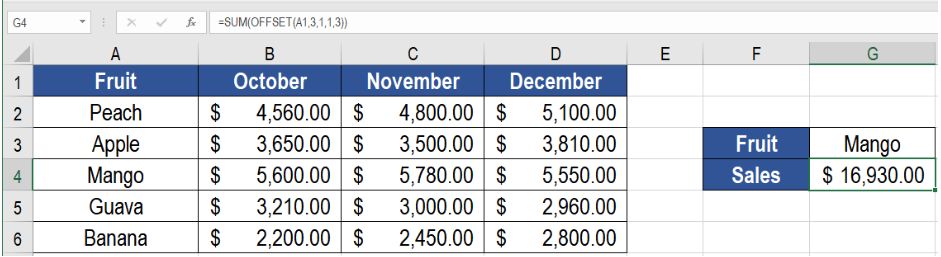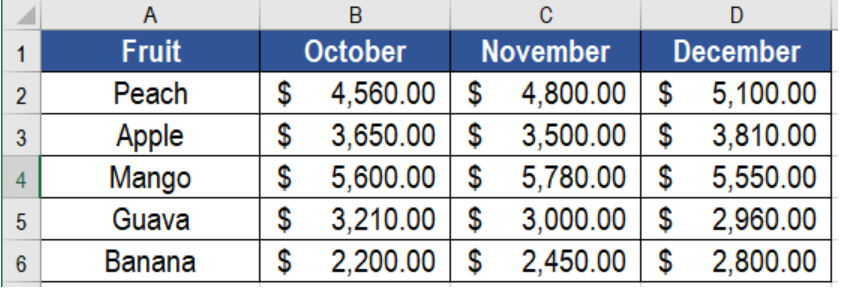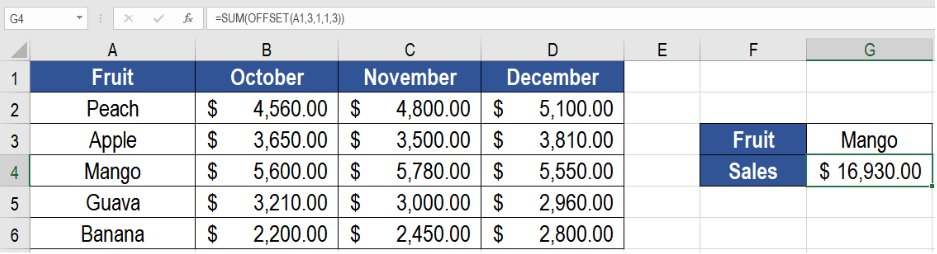Get instant live expert help with Excel or Google Sheets“My Excelchat expert helped me in less than 20 minutes, saving me what would have been 5 hours of work!”

#### Post your problem and you’ll get expert help in seconds.

Your message must be at least 40 characters
Our professional experts are available now. Your privacy is guaranteed.

# Excel OFFSET Function

Excel users often face the phenomenon of performing calculations on cells having a relative distance from other cells. In these situation, the OFFSET function comes in very handy. It returns a cell or range or cells that are a specified number of rows and columns away from a cell or range of cells. In this tutorial, we will learn how to use the offset function in Excel.Figure 1. Example of How to Use the Excel Offset Function

## Syntax

`=OFFSET (reference, rows, cols, [height], [width])`

## Arguments

• reference – This argument is required. It is a cell reference or range representing the starting point.
• rows – This argument is also required. It is the rows to offset below the starting reference.
• cols – This is a required argument as well. It represents the columns we want to offset right of the starting reference.
• height – This argument is optional. It is the height counted in rows of the reference that is returned.
• width – This argument is also optional. It represents the width calculated in columns of the reference returned.

## How the Formula Works

The OFFSET function in Excel returns a reference to a cell or range of cells offset from a starting point. This starting point can be a cell or a range of cells. The offset is supplied as rows or columns having a specified distance from the starting point. We can also provide the height and width arguments. They find the size of the reference which is created.

We can use OFFSET to create a dynamic named range for charts or pivot tables. This makes sure source data is always updated.

## Setting up Data

The following example uses a fruit sales database for the last quarter of 2018. Column A has the names, column B to D has the sales.Figure 2. The Sample Data

To find out the total sales information on oranges, we need to

• Go to cell G4. Select it with our mouse.
• Go to the formula bar of G4 and assign the formula `=SUM(OFFSET(A1,3,1,1,3))`.
• Press Enter.Figure 3. Applying the Formula

This will show the total sales of oranges in cell G4.

## Notes

1. The OFFSET function returns a reference only, it does not move any cells.
2. We can provide negative row and column numbers. It can reverse the normal offset direction of the row or columns. Negative columns offset to the left. Negative rows offset on the top.
3. OFFSET is recalculated whenever there is any change to a worksheet. It can slow down Excel in a complicated worksheet.
4. OFFSET will display the #REF! error value if the offset is outside the edge of the worksheet.
5. When height or width is omitted, the height and width of reference is used.
6. OFFSET can be used with any other function that expects to receive a reference.

Most of the time, the problem you will need to solve will be more complex than a simple application of a formula or function. If you want to save hours of research and frustration, try our live Excelchat service! Our Excel Experts are available 24/7 to answer any Excel question you may have. We guarantee a connection within 30 seconds and a customized solution within 20 minutes.

### Did this post not answer your question? Get a solution from connecting with the expert.Another blog reader asked this question today on Excelchat:
Solution examplesI need help fixing a Formula on Sheet 2, starting in Column G Row 22. In short, the formula should average the last six dollar amounts unless one of the last 6 dollar amounts is blank or 0. G56 on the same sheet works with the same logic but for only 3 months.
Solved by B. L. in 41 minsHello! There is a column with a certain number of rows in it. I need to calculate two averages: the average of every second row starting from the 1st one (aka the average of every odd-th cell) and the average of every second row starting from the 2nd one (aka the average of every even-th cell). I got tangled up with =OFFSET... Thanks!
Solved by C. J. in 37 minsI'm trying to use offset in a match function. My table is pulling data from a different tab called "operator runs". I want my first row to look at data in B1:B104 for "sample-A" and match "BA" from C1:C104 to pull data from column D. My second row, I want to look up text from F1:F104 and match "BA" from G1:G104 and pull data from column H and so on, offset by 4 columns to the right each time. This is my current function. I don't know how to implement the offset function in this complicated function. =MATCH(1,('Operator runs'!\$B\$1:\$B\$104="Sample-A")*('Operator runs'!\$C\$1:\$C\$104="BA"),0)
Solved by O. L. in 40 minsI'm looking to offset from =today(), for example: If cell A13=today(), display cell A20. If cell B13=today(), display cell B20. If cell C13=today(), display cell C20. I want to do this for 365 rows. Is there a simpler way of doing this than a huge nested IF statement?
Solved by K. H. in 34 minsHi, I need a formula to work out how many values are less than a certain time value, simple "countif" do not work as it is a filtered spreadsheet. Here's the formula that I have got so far: SUMPRODUCT(SUBTOTAL(3,OFFSET(Current_Month!N\$2:N\$10000,ROW(Current_Month!N\$2:N\$10000)-MIN(ROW(Current_Month!N\$2:N\$10000)),,1)),--(Current_Month!N\$2:N\$10000>"24:0:0")
Solved by K. L. in 30 mins## Subscribe to Excelchat.coAnother blog reader asked this question today on Excelchat: